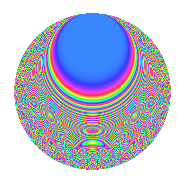Properties

 Label 6041.2.a.eLevel 6041 Weight 2 Character orbit 6041.a Self dual Yes Analytic conductor 48.238 Analytic rank 0 Dimension 112 CM No

Related objects

Newspace parameters

 Level: $$N$$ = $$6041 = 7 \cdot 863$$ Weight: $$k$$ = $$2$$ Character orbit: $$[\chi]$$ = 6041.a (trivial)

Newform invariants

 Self dual: Yes Analytic conductor: $$48.2376278611$$ Analytic rank: $$0$$ Dimension: $$112$$ Fricke sign: $$-1$$ Sato-Tate group: $\mathrm{SU}(2)$

$q$-expansion

The dimension is sufficiently large that we do not compute an algebraic $$q$$-expansion, but we have computed the trace expansion.

 $$\operatorname{Tr}(f)(q) =$$ $$112q$$ $$\mathstrut -\mathstrut 3q^{2}$$ $$\mathstrut +\mathstrut 14q^{3}$$ $$\mathstrut +\mathstrut 131q^{4}$$ $$\mathstrut +\mathstrut 13q^{5}$$ $$\mathstrut +\mathstrut 18q^{6}$$ $$\mathstrut -\mathstrut 112q^{7}$$ $$\mathstrut -\mathstrut 9q^{8}$$ $$\mathstrut +\mathstrut 116q^{9}$$ $$\mathstrut +\mathstrut O(q^{10})$$ $$\operatorname{Tr}(f)(q) =$$ $$112q$$ $$\mathstrut -\mathstrut 3q^{2}$$ $$\mathstrut +\mathstrut 14q^{3}$$ $$\mathstrut +\mathstrut 131q^{4}$$ $$\mathstrut +\mathstrut 13q^{5}$$ $$\mathstrut +\mathstrut 18q^{6}$$ $$\mathstrut -\mathstrut 112q^{7}$$ $$\mathstrut -\mathstrut 9q^{8}$$ $$\mathstrut +\mathstrut 116q^{9}$$ $$\mathstrut +\mathstrut 32q^{10}$$ $$\mathstrut +\mathstrut 14q^{11}$$ $$\mathstrut +\mathstrut 36q^{12}$$ $$\mathstrut +\mathstrut 22q^{13}$$ $$\mathstrut +\mathstrut 3q^{14}$$ $$\mathstrut +\mathstrut 19q^{15}$$ $$\mathstrut +\mathstrut 169q^{16}$$ $$\mathstrut +\mathstrut 11q^{17}$$ $$\mathstrut -\mathstrut 18q^{18}$$ $$\mathstrut +\mathstrut 52q^{19}$$ $$\mathstrut +\mathstrut 40q^{20}$$ $$\mathstrut -\mathstrut 14q^{21}$$ $$\mathstrut +\mathstrut 16q^{22}$$ $$\mathstrut +\mathstrut 38q^{23}$$ $$\mathstrut +\mathstrut 64q^{24}$$ $$\mathstrut +\mathstrut 99q^{25}$$ $$\mathstrut +\mathstrut 45q^{26}$$ $$\mathstrut +\mathstrut 65q^{27}$$ $$\mathstrut -\mathstrut 131q^{28}$$ $$\mathstrut +\mathstrut 10q^{29}$$ $$\mathstrut +\mathstrut q^{30}$$ $$\mathstrut +\mathstrut 133q^{31}$$ $$\mathstrut -\mathstrut 26q^{32}$$ $$\mathstrut +\mathstrut 27q^{33}$$ $$\mathstrut +\mathstrut 52q^{34}$$ $$\mathstrut -\mathstrut 13q^{35}$$ $$\mathstrut +\mathstrut 183q^{36}$$ $$\mathstrut -\mathstrut 13q^{37}$$ $$\mathstrut +\mathstrut 20q^{38}$$ $$\mathstrut +\mathstrut 74q^{39}$$ $$\mathstrut +\mathstrut 92q^{40}$$ $$\mathstrut +\mathstrut 25q^{41}$$ $$\mathstrut -\mathstrut 18q^{42}$$ $$\mathstrut -\mathstrut 11q^{43}$$ $$\mathstrut +\mathstrut 16q^{44}$$ $$\mathstrut +\mathstrut 63q^{45}$$ $$\mathstrut +\mathstrut 28q^{46}$$ $$\mathstrut +\mathstrut 71q^{47}$$ $$\mathstrut +\mathstrut 70q^{48}$$ $$\mathstrut +\mathstrut 112q^{49}$$ $$\mathstrut +\mathstrut 5q^{50}$$ $$\mathstrut +\mathstrut 57q^{51}$$ $$\mathstrut +\mathstrut 79q^{52}$$ $$\mathstrut -\mathstrut 10q^{53}$$ $$\mathstrut +\mathstrut 75q^{54}$$ $$\mathstrut +\mathstrut 146q^{55}$$ $$\mathstrut +\mathstrut 9q^{56}$$ $$\mathstrut -\mathstrut 83q^{57}$$ $$\mathstrut -\mathstrut 19q^{58}$$ $$\mathstrut +\mathstrut 56q^{59}$$ $$\mathstrut -\mathstrut 3q^{60}$$ $$\mathstrut +\mathstrut 80q^{61}$$ $$\mathstrut +\mathstrut 42q^{62}$$ $$\mathstrut -\mathstrut 116q^{63}$$ $$\mathstrut +\mathstrut 263q^{64}$$ $$\mathstrut -\mathstrut 26q^{65}$$ $$\mathstrut +\mathstrut 48q^{66}$$ $$\mathstrut +\mathstrut 29q^{67}$$ $$\mathstrut +\mathstrut 57q^{68}$$ $$\mathstrut +\mathstrut 56q^{69}$$ $$\mathstrut -\mathstrut 32q^{70}$$ $$\mathstrut +\mathstrut 100q^{71}$$ $$\mathstrut -\mathstrut 62q^{72}$$ $$\mathstrut +\mathstrut 73q^{73}$$ $$\mathstrut +\mathstrut 24q^{74}$$ $$\mathstrut +\mathstrut 89q^{75}$$ $$\mathstrut +\mathstrut 155q^{76}$$ $$\mathstrut -\mathstrut 14q^{77}$$ $$\mathstrut +\mathstrut 33q^{78}$$ $$\mathstrut +\mathstrut 140q^{79}$$ $$\mathstrut +\mathstrut 80q^{80}$$ $$\mathstrut +\mathstrut 120q^{81}$$ $$\mathstrut +\mathstrut 114q^{82}$$ $$\mathstrut +\mathstrut 36q^{83}$$ $$\mathstrut -\mathstrut 36q^{84}$$ $$\mathstrut -\mathstrut 2q^{85}$$ $$\mathstrut +\mathstrut 12q^{86}$$ $$\mathstrut +\mathstrut 96q^{87}$$ $$\mathstrut +\mathstrut 29q^{88}$$ $$\mathstrut +\mathstrut 47q^{89}$$ $$\mathstrut +\mathstrut 52q^{90}$$ $$\mathstrut -\mathstrut 22q^{91}$$ $$\mathstrut +\mathstrut 81q^{92}$$ $$\mathstrut -\mathstrut 10q^{93}$$ $$\mathstrut +\mathstrut 127q^{94}$$ $$\mathstrut +\mathstrut 96q^{95}$$ $$\mathstrut +\mathstrut 175q^{96}$$ $$\mathstrut +\mathstrut 80q^{97}$$ $$\mathstrut -\mathstrut 3q^{98}$$ $$\mathstrut +\mathstrut 74q^{99}$$ $$\mathstrut +\mathstrut O(q^{100})$$

Embeddings

For each embedding $$\iota_m$$ of the coefficient field, the values $$\iota_m(a_n)$$ are shown below.

For more information on an embedded modular form you can click on its label.

Label $$a_{2}$$ $$a_{3}$$ $$a_{4}$$ $$a_{5}$$ $$a_{6}$$ $$a_{7}$$ $$a_{8}$$ $$a_{9}$$ $$a_{10}$$
1.1 −2.81373 −3.44120 5.91707 1.30649 9.68259 −1.00000 −11.0216 8.84183 −3.67611
1.2 −2.80869 2.13107 5.88877 −3.52544 −5.98552 −1.00000 −10.9224 1.54145 9.90189
1.3 −2.77254 −2.14289 5.68701 −0.156494 5.94125 −1.00000 −10.2224 1.59196 0.433887
1.4 −2.76309 2.60057 5.63466 −1.29315 −7.18561 −1.00000 −10.0429 3.76297 3.57310
1.5 −2.71246 −0.244067 5.35744 −2.10613 0.662022 −1.00000 −9.10692 −2.94043 5.71281
1.6 −2.71151 1.27956 5.35230 1.05589 −3.46954 −1.00000 −9.08979 −1.36273 −2.86306
1.7 −2.67889 −2.12545 5.17645 −3.74850 5.69384 −1.00000 −8.50937 1.51753 10.0418
1.8 −2.57911 −0.702301 4.65183 2.85376 1.81131 −1.00000 −6.83937 −2.50677 −7.36016
1.9 −2.52776 3.15958 4.38957 3.22941 −7.98667 −1.00000 −6.04026 6.98297 −8.16316
1.10 −2.46248 −0.0194781 4.06380 −1.44141 0.0479644 −1.00000 −5.08207 −2.99962 3.54944
1.11 −2.45673 3.01946 4.03553 −1.39061 −7.41801 −1.00000 −5.00075 6.11715 3.41635
1.12 −2.43414 −1.84866 3.92503 3.83434 4.49989 −1.00000 −4.68578 0.417546 −9.33330
1.13 −2.41077 2.26439 3.81183 4.38346 −5.45893 −1.00000 −4.36791 2.12745 −10.5675
1.14 −2.37296 0.810716 3.63095 0.528258 −1.92380 −1.00000 −3.87017 −2.34274 −1.25354
1.15 −2.27713 −2.93009 3.18534 −2.32769 6.67221 −1.00000 −2.69918 5.58544 5.30045
1.16 −2.25493 0.275101 3.08473 2.79647 −0.620335 −1.00000 −2.44598 −2.92432 −6.30585
1.17 −2.22590 −2.92856 2.95465 1.01796 6.51870 −1.00000 −2.12496 5.57647 −2.26588
1.18 −2.13001 −1.53283 2.53693 −2.08639 3.26494 −1.00000 −1.14367 −0.650428 4.44403
1.19 −2.10602 −0.719393 2.43533 1.34496 1.51506 −1.00000 −0.916817 −2.48247 −2.83252
1.20 −2.06364 −0.217047 2.25861 3.48472 0.447908 −1.00000 −0.533675 −2.95289 −7.19121
See next 80 embeddings (of 112 total)
 $$n$$: e.g. 2-40 or 990-1000 Embeddings: e.g. 1-3 or 1.112 Significant digits: Format: Complex embeddings Normalized embeddings Satake parameters Satake angles

Inner twists

This newform does not have CM; other inner twists have not been computed.

Atkin-Lehner signs

$$p$$ Sign
$$7$$ $$1$$
$$863$$ $$-1$$

Hecke kernels

This newform can be constructed as the kernel of the linear operator $$T_{2}^{112} + \cdots$$ acting on $$S_{2}^{\mathrm{new}}(\Gamma_0(6041))$$.